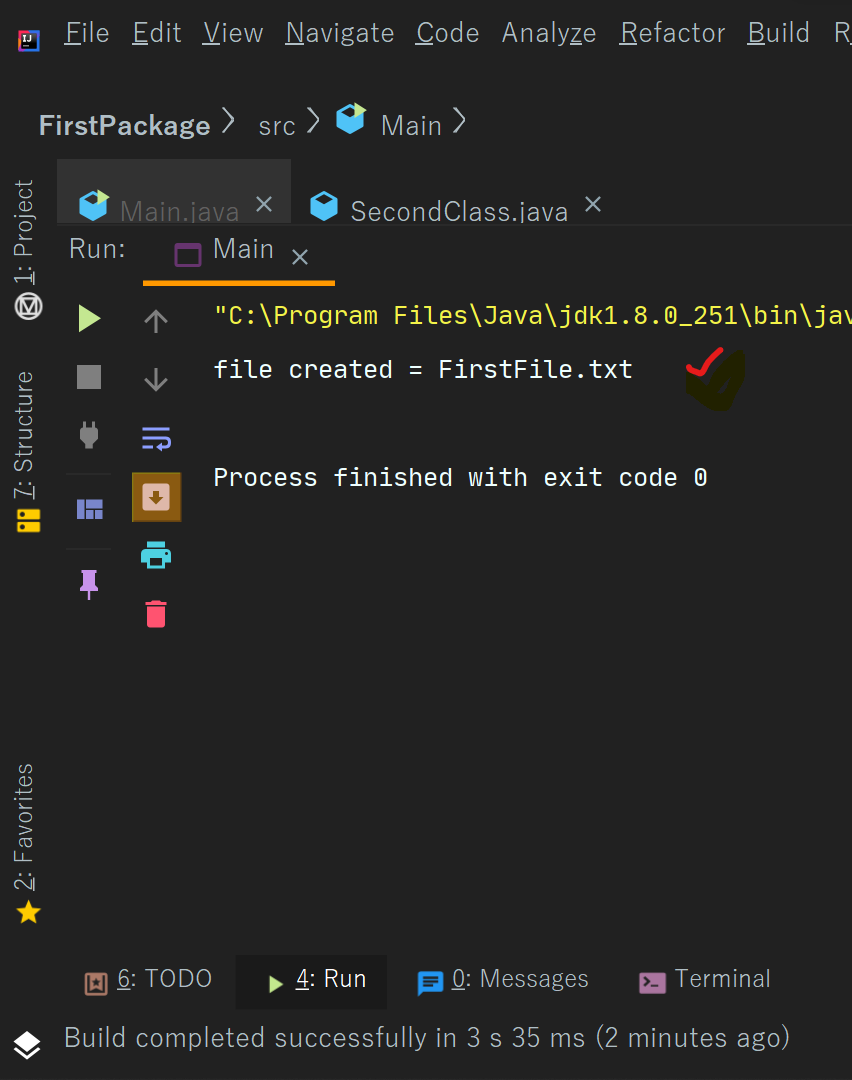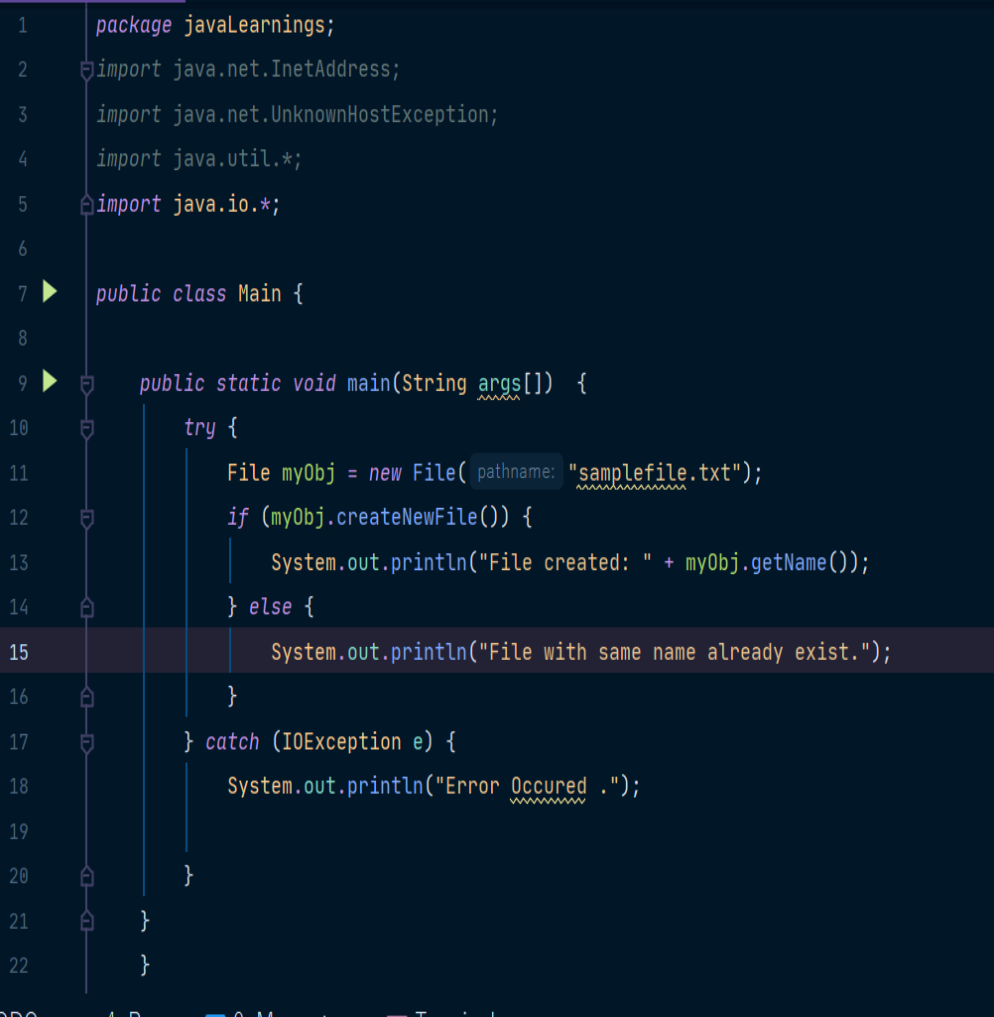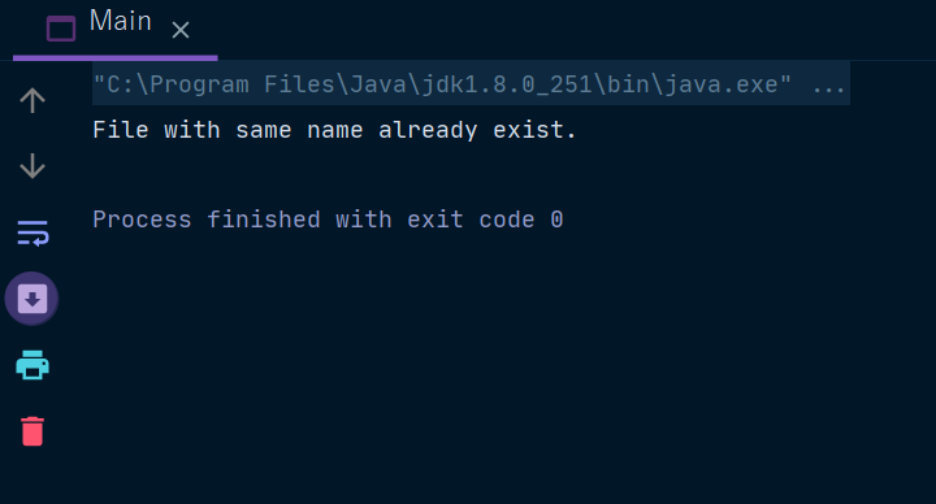Java Logical Operators

# Java Logical Operators

Logical Operator

Combine two boolean expression, and as a result return true, or false.
These operators are used to perform logical “AND”, “OR” and “NOT” operation, i.e. the function similar to AND gate and OR gate in digital electronics.
They are used to combine two or more conditions/constraints or to complement the evaluation of the original condition under particular consideration.
One thing to keep in mind is the second condition is not evaluated if the first one is false, i.e. it has a short-circuiting effect.

It is used extensively to test for several conditions for making decision. Let’s look at each of the logical operators in a detailed manner.
In other words, we have three types of logical operators -

1. Logical AND (&&)

2. Logical OR (||)

3. Logical NOT (!)

1. Logical AND
This operator return true only when both the expressions are true, means non- zero.

Syntax-

 condition1 && condition2

2. Logical OR
This operator returns true when anyone expression is true.

3. Logical NOT
This operator return opposite value of expression i.e., !(true) = false, and vice versa.

 import java.util.*; class Bool { public static void main(String[] args) { Random rand = new Random(47); int i = rand.nextInt(100); int j = rand.nextInt(100); //! print("i && j is " + (i && j)); //! print("i || j is " + (i || j)); //! print("!i is " + !i); print("(i < 10) && (j < 10) is " + ((i < 10) && (j < 10)) ); print("(i < 10) || (j < 10) is " + ((i < 10) || (j < 10)) ); }   Output (i < 10) && (j < 10) is false (i < 10) || (j < 10) is false

Examples To Create A File In Java
We can create file using createNewFile() method which is found in File class .
We will understand it with the help of an example -

Example 1 -

 import second.*; import java.io.File; import java.io.IOException; import java.util.*; // Post decrement operator class Main{   public static void main(String[] args) {   try{       File ob = new File("FirstFile.txt"); if (ob.createNewFile())   System.out.println("file created = "+ob.getName()); else   System.out.println("File with the same name already exists = ");   }   catch (IOException exception){ System.out.println("Error Occured = "+exception);   }       }   }

Output -Example  2  -Output -  one sample output would be -